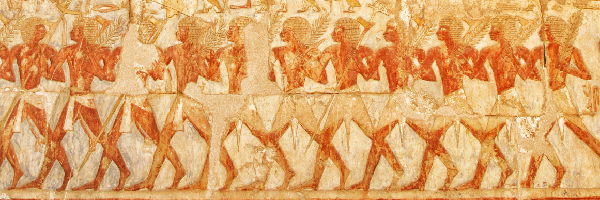# Egyptian Fractions - Writing them allHow can we write a fraction with a large numerator, such as $\frac{115}{137}$, as an Egyptian Fraction?

One way might be to write $\frac{115}{137}=\frac{1}{137}+\frac{1}{137}+...+\frac{1}{137}$ and try to find lots of different ways to write $\frac{1}{137}$ as a sum of unit fractions...

I wonder if there's a more efficient way?

One possibility is to try a so-called Greedy Algorithm:

At each stage, write down the largest possible unit fraction that is smaller than the fraction you're working on.

For example, let's start with $\frac{11}{12}$.

The largest possible unit fraction that is smaller than $\frac{11}{12}$ is $\frac{1}{2}$.

$\frac{11}{12} -\frac{1}{2}=\frac{5}{12}$

So $\frac{11}{12}=\frac{1}{2}+\frac{5}{12}$

The largest possible unit fraction that is smaller than $\frac{5}{12}$ is $\frac{1}{3}$

$\frac{5}{12} -\frac{1}{3}=\frac{1}{12}$

So $\frac{11}{12}=\frac{1}{2}+\frac{1}{3}+\frac{1}{12}$

Choose some fractions of your own and apply the Greedy Algorithm until you are left with a sum of unit fractions.

Can you find any fractions where the Greedy Algorithm only takes a small number of steps?

Can you find any fractions where the Greedy Algorithm takes a very large number of steps?

Are there any fractions where the Greedy Algorithm doesn't terminate? How do you know?